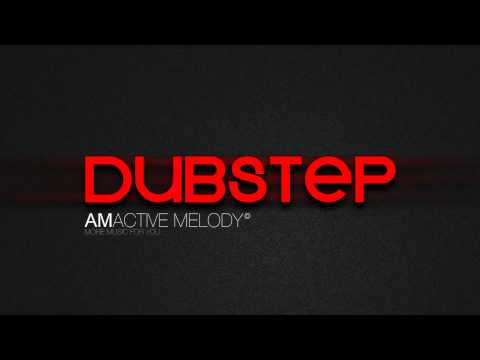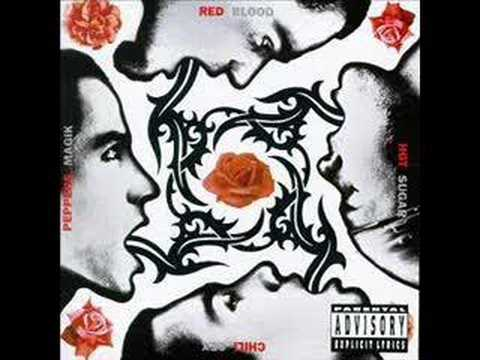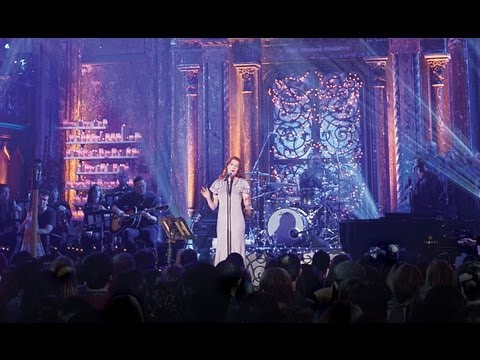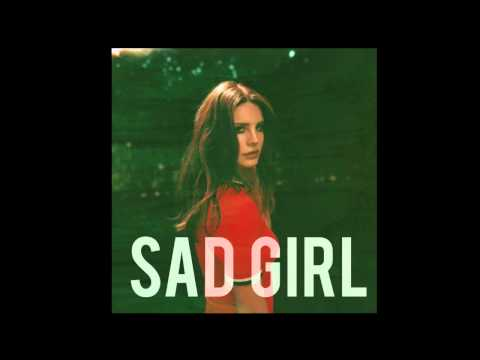Lyrics

Is this the point where we give up? Is this the point where we give in? Is this the point where we turn ourselves in? Is this the time to ask questions? Is this the time to seek answers? Is this the time to throw it all in? When enough is enough that's when you know that you're halfway there When enough is enough that's when you know that you're halfway there You're halfway there That's when you know that you're halfway there This is the point where we stand up This is the point where we take back The things that are keeping us from being free When enough is enough that's when you know that you're halfway there When enough is enough that's when you know that you're halfway there That's when you know that you're halfway there Your voice the airwaves sent you prayed my life would end, The wasted time I spent ashamed I called you friend, Give me one reason, I need a reason, one reason

Suggestions Song

Autoplay